Next: Kelvin Circulation Theorem Up: Incompressible Inviscid Flow Previous: Vortex Lines, Vortex Tubes,

# Circulation and Vorticity

Consider a closed curve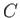situated entirely within a moving fluid. The vector line integral (see Section A.14)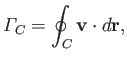(4.76)

where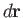is an element of, and the integral is taken around the whole curve, is termed the circulation of the flow around the curve. The sense of circulation (i.e., either clockwise or counter-clockwise) is arbitrary.

Letbe a surface having the closed curvefor a boundary, and letbe an element of this surface (see Section A.7) with that direction of the normal which is related to the chosen sense of circulation aroundby the right-hand circulation rule. (See Section A.8.) According to the curl theorem (see Section A.22),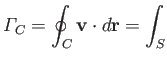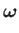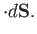(4.77)

Thus, we conclude that circulation and vorticity are intimately related to one another. In fact, according to the previous expression, the circulation of the fluid around loopis equal to the net sum of the intensities of the vortex filaments passing through the loop and piercing the surface(with a filament making a positive, or negative, contribution to the sum depending on whether it pierces the surface in the direction determined by the chosen sense of circulation aroundand the right-hand circulation rule, or in the opposite direction). One important proviso to Equation (4.77) is that the surfacemust lie entirely within the fluid.Next: Kelvin Circulation Theorem Up: Incompressible Inviscid Flow Previous: Vortex Lines, Vortex Tubes,
Richard Fitzpatrick 2016-03-31